# 17.5: Nucleophilic Substitution with Enolate Anions

$$\newcommand{\vecs}{\overset { \rightharpoonup} {\mathbf{#1}} }$$ $$\newcommand{\vecd}{\overset{-\!-\!\rightharpoonup}{\vphantom{a}\smash {#1}}}$$$$\newcommand{\id}{\mathrm{id}}$$ $$\newcommand{\Span}{\mathrm{span}}$$ $$\newcommand{\kernel}{\mathrm{null}\,}$$ $$\newcommand{\range}{\mathrm{range}\,}$$ $$\newcommand{\RealPart}{\mathrm{Re}}$$ $$\newcommand{\ImaginaryPart}{\mathrm{Im}}$$ $$\newcommand{\Argument}{\mathrm{Arg}}$$ $$\newcommand{\norm}{\| #1 \|}$$ $$\newcommand{\inner}{\langle #1, #2 \rangle}$$ $$\newcommand{\Span}{\mathrm{span}}$$ $$\newcommand{\id}{\mathrm{id}}$$ $$\newcommand{\Span}{\mathrm{span}}$$ $$\newcommand{\kernel}{\mathrm{null}\,}$$ $$\newcommand{\range}{\mathrm{range}\,}$$ $$\newcommand{\RealPart}{\mathrm{Re}}$$ $$\newcommand{\ImaginaryPart}{\mathrm{Im}}$$ $$\newcommand{\Argument}{\mathrm{Arg}}$$ $$\newcommand{\norm}{\| #1 \|}$$ $$\newcommand{\inner}{\langle #1, #2 \rangle}$$ $$\newcommand{\Span}{\mathrm{span}}$$

## Alkylation of Ketones

The synthetic chemistry of enolate anions is centered on their nucleophilic and basic properties. Accordingly these ions participate in $$S_\text{N}2$$ reactions with suitable alkyl compounds:However, there are a number of complicating factors to consider. First, the basic conditions needed to form the enolate ions often lead to side reactions such as aldol addition and $$E2$$ elimination of $$\ce{RX}$$ compounds. Aldol addition is minimized if the carbonyl compound is a ketone with a structure unfavorable for aldol addition or if all of the carbonyl compound is converted to its enolate. To convert all of a simple carbonyl to its enolate usually requires a very strong base, such as $$\ce{NH_2^+}$$ in an aprotic solvent or liquid ammonia. Because the enolate anion itself is a strong base, best results are obtained when the halide, $$\ce{RX}$$, does not undergo $$E2$$ reactions readily.

The second complication arises if the alkyl compound reacts with both carbon and oxygen of the nucleophilic enolate anion. The carbon product is the result of "$$\ce{C}$$-alkylation", whereas the oxygen product is the result of "$$\ce{O}$$-alkylation":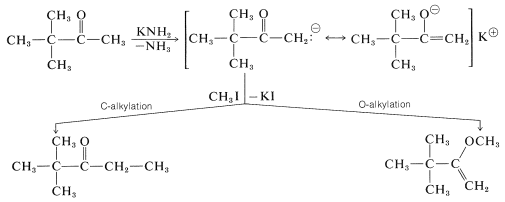The possibility of the enolate anion acting as if its charge were effectively concentrated on carbon or on oxygen was discussed previously in connection with aldol addition (Section 17-3B). However, the situation there was quite different from the one here, because aldol addition is easily reversible, whereas alkylation is not. Furthermore, while the aldol reaction involving $$\ce{C-O}$$ bond formation is unfavorable $$\left( \Delta H^0 = + 20 \: \text{kcal mol}^{-1} \right)$$ compared to $$\ce{C-C}$$ bond formation $$\left( \Delta H^0 = - 4 \: \text{kcal mol}^{-1} \right)$$, both $$\ce{O}$$- and $$\ce{C}$$-alkylation of the anion have $$\Delta H^0 < 0$$.

Whether $$\ce{C}$$- or $$\ce{O}$$-alkylation predominates depends on kinetic control (Section 10-4A). It is not a simple matter to predict which of the two positions of the enolate will be more nucleophilic, and in fact, mixtures of products often are obtained in distributions that depend on the solvent used, the temperature, the nature of $$\ce{X}$$, and the nature of the base employed to form the anion. $$\ce{O}$$-Alkylation tends to occur with ketones of high enol content (which usually means that the enolate anion will have especially high charge density on oxygen) and with alkylating agents possessing a high degree of $$S_\text{N}2$$ reactivity.

There is another correlation that seems to have validity in many situations, at least where kinetic control is dominant; namely, the freer (less associated) the ambident anions is from its cation, the more likely is the electrophile to attack the atom of the anion with the highest negative charge. Thus $$\ce{O}$$-alkylation of the sodium enolate of 2-propanone is favored in aprotic solvents that are good at solvating cations [such as $$\ce{(CH_3)_2SO}$$, Section 8-7F].

In the alkylation of unsymmetrical ketones, formation of more than one enolate anion is possible, and when this occurs, mixtures of products are obtained. Thus,However, when one of the possible enolate anions is especially stabilized, either by conjugation or by strong electron-withdrawing groups, that enolate usually is the dominant form and only one product is formed. Thus 2,4-pentanedione is methylated at $$\ce{C3}$$, not at $$\ce{C1}$$: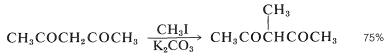## Alkylation of Enamines

Enamines (Section 16-4C), like enolate anions, have two reactive positions and, in principle, can give either $$\ce{N}$$- or $$\ce{C}$$-alkylation.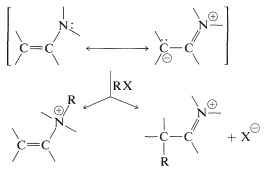Both products may be formed, but they can be separated readily because, on treatment with dilute acid, only the $$\ce{C}$$-alkylation product hydrolyzes to a ketone. Generally, the alkylated ketone is the desired product:Alkylation of enamines therefore is a feasible, and sometimes much more useful, alternative to the direct alkylation of ketones because it proceeds under less strongly basic conditions. The sequence starts with conversion of a ketone to an enamine,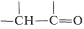$$\overset{\ce{RNH_2}}{\longrightarrow}$$$$+ \ce{H_2O}$$, followed by $$\ce{C}$$-alkylation of the enamine,$$\overset{\ce{RX}}{\longrightarrow}$$$$+ \ce{X}^\ominus$$, and ends with hydrolysis to the alkylated ketone,$$+ \ce{H_2O} \rightarrow$$$$+ \ce{R_2NH_2^-}$$. A typical example of the use of enamines for alkylation of a ketone follows:Several important biological reactions utilize enamine intermediates as carbon nucleophiles in $$\ce{C-C}$$ bond-forming reactions. One example is discussed in Section 17-3F.

## Alkylation of Sulfur-Stabilized Carbanions

The chemistry of carbanions stabilized by groups other than carbonyl functions is closely analogous to the chemistry of enolate anions. We have seen that $$\ce{C-H}$$ acidity of compounds with the structural featurecan be significant (p$$K_\text{a}$$ of 25 or less) when $$\ce{X}$$ is an atom or group that can effectively delocalize the negative charge on carbon in. Typical $$\ce{X}$$ groups are $$\ce{C=O}$$, $$\ce{C \equiv N}$$, $$\ce{PR_3^+}$$, $$\ce{SR_2^+}$$, $$\ce{SO_2R}$$, and $$\ce{SR}$$. Consequently, we can expect that carbanions of the type, when formed, will resemble enolate anions and will undergo addition reactions to $$\ce{C=O}$$ and $$\ce{C=C}$$, and will be alkylated with halides of good $$S_\text{N}2$$ reactivity. In fact, the reactions of ylides discussed in Section 16-4A are examples of the addition of phosphorus-, sulfur-, and nitrogen-stabilized carbanions to carbonyl groups.

Sulfur in its higher oxidation states (e.g., sulfone, $$\ce{-SO_2}-$$) is especially effective in stabilizing adjacent carbanion centers. However, from a synthetic standpoint there are disadvantages to the sulfone grouping in that the better stabilized carbanions also are the least reactive, and subsequent removal of the sulfone grouping can be difficult. A good balance between carbanion stability, carbanion reactivity, and ease of $$\ce{C-S}$$ bond cleavage is present in the structures $$\ce{RS-CH_2-SR}$$ and. This is illustrated below for a strikingly simple concept for preparing cyclobutanone, in which the ring carbons are derived from methanal and 1,3-dibromopropane: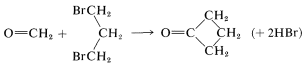To achieve this synthesis, the methanal first is converted to a thioketal, which then is partially oxidized to give $$13$$. Treatment of $$13$$ with a strong base converts it to the carbanion, which can be readily alkylated. By using 1,3-dibromopropane and two equivalents of base, a double displacement forms the cyclic product, $$14$$. The sulfur groups of $$14$$ can be removed easily by acid hydrolysis to give cyclobutanone: